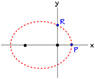# Parametric Equations of Keplerian Orbit

derravaragh

## Homework Statement

The figure illustrates a Keplerian orbit, with Cartesian coordinates (x,y) and
plane polar coordinates (r,φ).
F = -(G*M*m)/r^2
The parametric equations for the orbit:
r(ψ) = a ( 1 − e cos ψ)
tan(φ/2) = [(1+e)/(1-e)]^(1/2)* tan(ψ/2)
t(ψ) = (T/2π) ( ψ − e sin ψ)
where ψ is the independent, parametric variable; a, e, and T are constants.

Prove, directly from the parametric equations, that the angular momentum L is
constant. Express L in terms of the constants of the orbit (and any other relevant parameters).

L = r x p
τnet = r x F

## The Attempt at a Solution

I know that in order for L to be constant, its derivative (net torque) must be 0, but this isn't really getting me anywhere. I know torque = rxF and L = rmv, but quite frankly I can't seem to figure out how to do this using the parametric equations.
What I have done using the parametric equations is this:
L = m*r(ψ)*v(t) = m*(a*(1-e*cos(ψ)))*v(t)
From here I'm having a difficult time finding an expression for v(t). I tried using the relations
ω=((2*π)/T) and v = ω*r for the following work:
t(ψ) = (1/ω)*(ψ-esin(ψ))
==> ω = (ψ-esin(ψ))/t(ψ)
==> ω = (ψ-esin(ψ))/((T/(2π))*(ψ-esin(ψ))
==> ω = (2π/T) (this obviously backtracked)
==> v = r*(2π/T)

∴L = m*(a*(1-e*cos(ψ)))*(a*(1-e*cos(ψ)))*(2π/T)
or L = m*a2*(1-e*cos(ψ))2*(2π/T)

I'm pretty sure this isn't right because it isn't going to be constant for different r values. Any help would be appreciated.

#### Attachments

•c7bf0944-3182-4b94-9a48-5fbcae468dd0.png
1.5 KB · Views: 416
Last edited by a moderator:

Mentor
The time-derivative of L = r x p is not r x F. Don't forget that r depends on time as well.
L = rmv
Only if r and v are perpendicular to each other. In general, they are not.

derravaragh
Ok, well my intuition tells me to make L=r(psi)mvsin(θ) where θ is the angle between the positive x-axis and the line from the focal point to the point in the orbit

In my notes, we proved momentum was constant by making momentum L = m(x*vy - y*vx) and converting to polar coordinates. Does this equation apply to any elliptical orbit?

As for the time derivative of L = rmv, I am just unsure of how to do this as I can't seem to figure out the correct expression for v.

Last edited:
Mentor
If you calculate the cross-product, this is exactly what you get. It looks tricky to get v_y, v_x based on the functions in the problem statement, however. L=r(psi)mvsin(θ) looks easier to evaluate.

As for the time derivative of L = rmv, I am just unsure of how to do this as I can't seem to figure out the correct expression for v.
The formula is wrong for an elliptic orbit anyway, there is no need to get v.

Staff Emeritus
Homework Helper
The time-derivative of L = r x p is not r x F. Don't forget that r depends on time as well.
Yes, it is. When you apply the product rule, the other term drops out because dr/dt is parallel to p.

Mentor
Oh, right.
Good, now it makes sense again (as ##\dot{L}=\tau=r \times F##).

derravaragh
The formula is wrong for an elliptic orbit anyway, there is no need to get v.

Ok, I'm confused here. I'm continuing forward with L = mvr(ψ)sin(θ), but I don't understand why I don't need to get v, since v isn't constant? I know that when taking the time derivative of L one of the terms is r x p = 0 because they parallel

Staff Emeritus